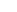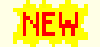# Aapo Hyvärinen: Publications

## Publications by topic## Estimation theory

[These papers propose principles for estimation of statistical models, especially non-normalized ones, a.k.a. energy-based models.]

#### Review on the topic

M. U. Gutmann and A. Hyvärinen. Estimation of unnormalized statistical models without numerical integration. Proc. Int. Workshop on Information-Theoretic Methods in Science and Engineering, Tokyo, Japan, 2013.
pdf

#### General

T. Matsuda, M. Uehara, and A. Hyvärinen. Information criteria for non-normalized models. JMLR, 22: 1-33, 2021
pdf

#### Noise-contrastive estimation

M. Gutmann and A. Hyvärinen. Noise-Contrastive Estimation of Unnormalized Statistical Models, with Applications to Natural Image Statistics, J. Machine Learning Research 13:307-361, 2012.
pdf  Matlab code
[One of our two fundamental methods for estimating statistical models when the normalization constant (partition function) is not known. Based on AISTATS2010 paper.]O. Chehab, A. Gramfort and A. Hyvärinen. Optimizing the Noise in Self-Supervised Learning: from Importance Sampling to Noise-Contrastive Estimation. Arxiv, Jan 2023.
pdf
[Analysis of how to choose the noise in noise-contrastive estimation from the viewpoint of statistical optimality.]

M. Pihlaja, M. Gutmann and A. Hyvärinen. A Family of Computationally Efficient and Simple Estimators for Unnormalized Statistical Models. Proc. UAI2010.
pdf
[Generalizes the method above and shows its connection to importance sampling. ]

M. Gutmann and A. Hyvärinen. Learning features by contrasting natural images with noise. Proc. Int. Conf. on Artificial Neural Networks (ICANN2009), Limassol, Cyprus, 2009.
pdf
[The very first paper on noise-contrastive estimation. Proposed it from a very intuitive viewpoint.]

#### Score matching

A. Hyvärinen. Estimation of non-normalized statistical models using score matching. Journal of Machine Learning Research, 6:695--709, 2005.
pdf  errata
[A computationally simple yet consistent method for estimating statistical models when the normalization constant (partition function) is not known. ]

A. Hyvärinen. Optimal approximation of signal priors. Neural Computation, 20:3087-3110, 2008.
pdf  gzipped ps
[Shows that the optimal method for estimating a prior model (e.g. of natural images) for Bayesian inference (e.g. denoising) is not maximum likelihood, but score matching and some of its generalizations.]

A. Hyvärinen. Some extensions of score matching. Computational Statistics & Data Analysis, 51:2499-2512, 2007.
pdf
[Extends score matching to binary data and non-negative data, and shows that the estimator can be obtained in closed form for exponential families.]

A. Hyvärinen. Connections between score matching, contrastive divergence, and pseudolikelihood for continuous-valued variables. IEEE Transactions on Neural Networks, 18(5):1529-1531, 2007.
pdf  gzipped ps
[Shows how score matching can be viewed as a deterministic first-order approximation of contrastive divergence.]

A. Hyvärinen. Estimation theory and information geometry based on denoising. Proc. Workshop on Information Theory in Science and Engineering, Tampere, Finland, 2008.
pdf
[A short review of the theory of score matching, although from a very abstract viewpoint.]